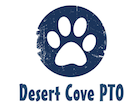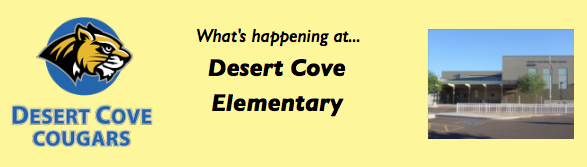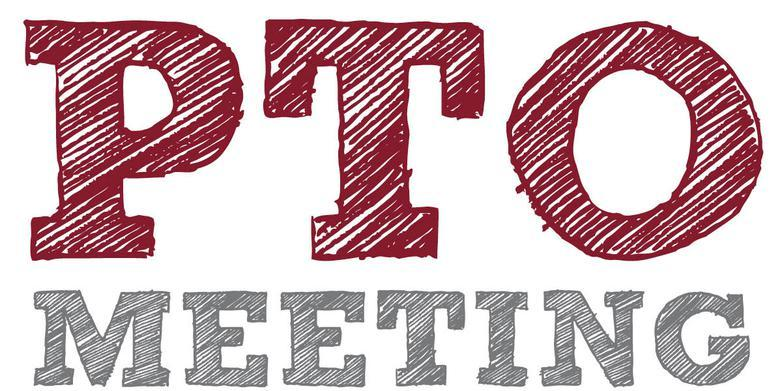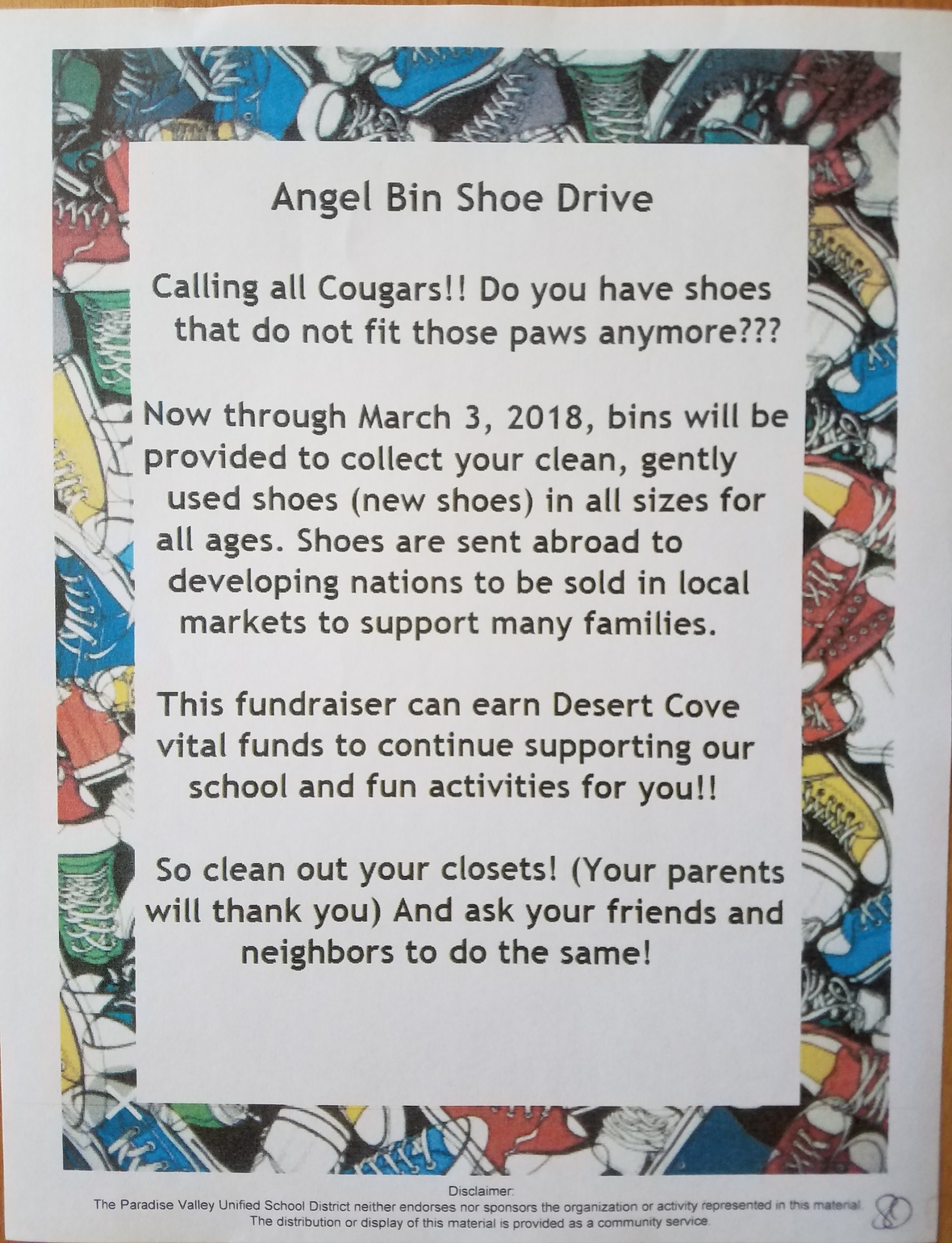/* styles */ Hi DC Community!# The Desert Cove eNews will now be sent on a MONTHLY basis instead of weekly.DECEMBER

▪ Dec 1: Polar Express PJ Night at Barnes & Noble
▪ Dec 5: EARLY RELEASE
▪ Dec 8: PDQ Restaurant Night
▪ Dec 12: PTO Meeting @ 6pm
▪ Dec 21: Teacher/Staff Holiday Luncheon
▪ Dec 25 - Jan 5: WINTER BREAK
▪ Ongoing: Angel Bin Shoe Drive
 ▪ Dec 1: Polar Express PJ Night at Barnes & Noble
 ▪ Dec 5: EARLY RELEASE
 ▪ Dec 8: PDQ Restaurant Night
 ▪ Dec 12: PTO Meeting @ 6pm
 ▪ Dec 21: Teacher/Staff Holiday Luncheon
 ▪ Dec 25 - Jan 5: WINTER BREAK
 ▪ Ongoing: Angel Bin Shoe Drive

 table div table+table+table+table+table+table+table div table{width:100%;padding:0}table div table+table+table+table+table+table+table div table img{width:96.23%;padding:0;float:none}table div table+table+table+table+table+table+table div table td{width:100%;padding:0 1.88% 18px}/* styles */## Polar Express PJ Night is TONIGHT!## Free Gift wrapping provided by our Student Council (STUCO)

 table div table+table+table+table+table+table+table+table+table+table+table div table{width:100%;padding:0}table div table+table+table+table+table+table+table+table+table+table+table div table img{width:96.23%;padding:0;float:none}table div table+table+table+table+table+table+table+table+table+table+table div table td{width:100%;padding:0 1.88% 18px}/* styles */## PDQ Restaurant Night - Dec 8thtable div table+table+table+table+table+table+table+table+table+table+table+table+table+table div table{width:100%;padding:0}table div table+table+table+table+table+table+table+table+table+table+table+table+table+table div table img{width:96.23%;padding:0;float:none}table div table+table+table+table+table+table+table+table+table+table+table+table+table+table div table td{width:100%;padding:0 1.88% 18px}/* styles */# Next PTO Meeting is Tuesday, December 12th!

## Can't make it? Meeting Minutes are posted on our website - Click Here

 table div table+table+table+table+table+table+table+table+table+table+table+table+table+table+table+table+table+table div table{width:100%;padding:0}table div table+table+table+table+table+table+table+table+table+table+table+table+table+table+table+table+table+table div table img{width:96.23%;padding:0;float:none}table div table+table+table+table+table+table+table+table+table+table+table+table+table+table+table+table+table+table div table td{width:100%;padding:0 1.88% 18px}/* styles */table div table+table+table+table+table+table+table+table+table+table+table+table+table+table+table+table+table+table+table+table div table{width:100%;padding:0}table div table+table+table+table+table+table+table+table+table+table+table+table+table+table+table+table+table+table+table+table div table img{width:96.23%;padding:0;float:none}table div table+table+table+table+table+table+table+table+table+table+table+table+table+table+table+table+table+table+table+table div table td{width:100%;padding:0 1.88% 18px}/* styles */table div table+table+table+table+table+table+table+table+table+table+table+table+table+table+table+table+table+table+table+table+table div table td,table.module-20{width:100%;padding:0}table div table+table+table+table+table+table+table+table+table+table+table+table+table+table+table+table+table+table+table+table+table div table{width:100%;float:none;margin-left:auto;margin-right:auto;padding:0}table div table+table+table+table+table+table+table+table+table+table+table+table+table+table+table+table+table+table+table+table+table div table a{border:0 none;text-decoration:none}table div table+table+table+table+table+table+table+table+table+table+table+table+table+table+table+table+table+table+table+table+table div table img{width:100%!important;border:0 none;text-decoration:none}/* styles */

# All important information regarding PTO News & Events will be on the DESERT COVE PTO WEBSITE(click to open)

 /* styles */ You can also subscribe to more frequent updates through:We have created a page on the PTO website where you can access the following communications:

Desert Cove PTO eNews archives
Desert Cove Principal News
PV School District eNews
United Parent Council eNews